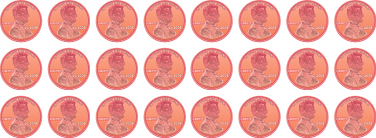## Notation and Modeling Multiplication of Whole Numbers

### Learning Outcomes

• Use symbols and words to represent multiplication
• Model multiplication of whole numbers

## Use Multiplication Notation

Suppose you were asked to count all these pennies shown below.Would you count the pennies individually? Or would you count the number of pennies in each row and add that number $3$ times.

$8+8+8$

Multiplication is a way to represent repeated addition. So instead of adding $8$ three times, we could write a multiplication expression.

$3\times 8$

We call each number being multiplied a factor and the result the product. We read $3\times 8$ as three times eight, and the result as the product of three and eight.

There are several symbols that represent multiplication. These include the symbol $\times$ as well as the dot, $\cdot$ , and parentheses $\left(\right)$

### Operation Symbols for Multiplication

To describe multiplication, we can use symbols and words.

Operation Notation Expression Read as Result
$\text{Multiplication}$ $\times$

$\cdot$

$\left(\right)$

$3\times 8$

$3\cdot 8$

$3\left(8\right)$

$\text{three times eight}$ $\text{the product of 3 and 8}$

### example

Translate from math notation to words:

1. $7\times 6$
2. $12\cdot 14$
3. $6\left(13\right)$

Solution

• We read this as seven times six and the result is the product of seven and six.
• We read this as twelve times fourteen and the result is the product of twelve and fourteen.
• We read this as six times thirteen and the result is the product of six and thirteen.

### try it

In the video below we present more ways that multiplication is expressed with words, and how to translate the words to math notation.

## Model Multiplication of Whole Numbers

There are many ways to model multiplication. Unlike in the previous sections where we used $\text{base - 10}$ blocks, here we will use counters to help us understand the meaning of multiplication. A counter is any object that can be used for counting. We will use round blue counters.

### example

Model: $3\times 8$.

### try it

Model each multiplication: $4\times 6$

Model each multiplication: $5\times 7$